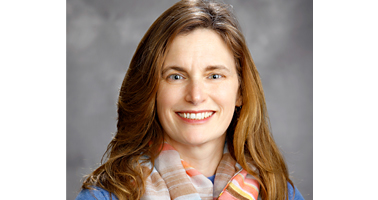Past Event: Oden Institute Seminar

# Generalized Tensor Decomposition: Utility for Data Analysis and Mathematical Challenges

Tamara G. Kolda, Mathematical Consultant, MathSci.ai based in California.

3:30 – 5PM
Tuesday Feb 7, 2023

POB 6.304 & Zoom

## Abstract

Tensor decomposition is an unsupervised learning methodology that has applications in a wide variety of domains, including chemometrics, criminology, and neuroscience. We focus on low-rank tensor decomposition using  canonical polyadic or CANDECOMP/PARAFAC format. A low-rank tensor decomposition is the minimizer according to some nonlinear program. The usual objective function is the sum of squares error (SSE) comparing the data tensor and the low-rank model tensor. This leads to a nicely-structured problem with subproblems that are linear least squares problems which can be solved efficiently in closed form. However, the SSE metric is not always ideal. Thus, we consider using other objective functions. For instance, KL divergence is an alternative metric is useful for count data and results in a nonnegative factorization. In the context of nonnegative matrix factorization, for instance, KL divergence was popularized by Lee and Seung (1999). We can also consider various objectives such as logistic odds for binary data, beta-divergence for nonnegative data, and so on. We show the benefits of altenative objective functions on real-world data sets. We consider the computational of generalized tensor decomposition based on other objective functions, summarize the work that has been done thus far, and illuminate open problems and challenges.

## Biography

Tamara Kolda is an independent mathematical consultant under the auspices of her company MathSci.ai based in California. She specializes in mathematical algorithms and computation methods for tensor decompositions, tensor eigenvalues, graph algorithms, randomized algorithms, machine learning, network science, numerical optimization, and distributed and parallel computing. She is a member of the National Academy of Engineering (NAE), Fellow of the Society for Industrial and Applied Mathematics (SIAM), and Fellow of the Association for Computing Machinery (ACM).  For more information, see: https://www.mathsci.ai/### Event information

Date
3:30 – 5PM
Tuesday Feb 7, 2023
Location POB 6.304 & Zoom
Hosted by Per-Gunnar Martinsson